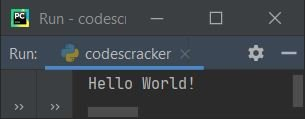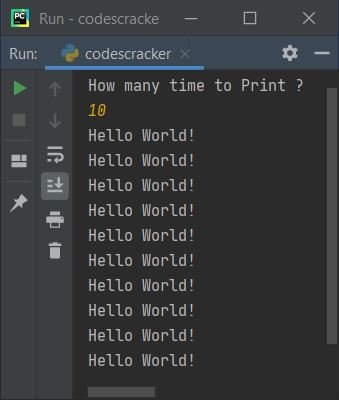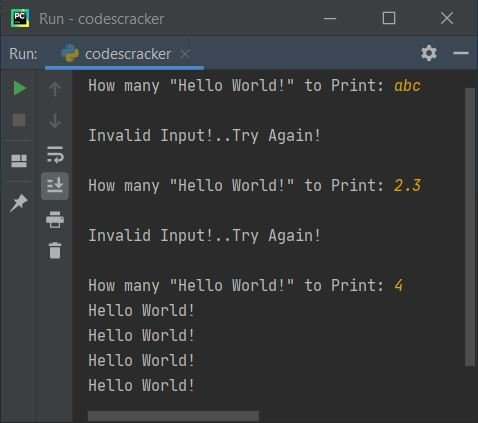# Python Program to Print Hello World

In this article, I've created some programs in Python, to print Hello World. Here are the list of Hello World programs in Python:

• Simplest Hello World Program
• Print Hello World 5 times using for Loop
• Print Hello World n times. The value of n must be entered by user
• Print Hello World using Function
• Using Class and Object

## Simplest Hello World Program in Python

To print "Hello World" in Python, use print() statement, and place "Hello World" inside it like done in the program given below. The question is, write a Python program to print Hello World!. Here is its answer:

`print("Hello World!")`

This Python program produces output as shown in the snapshot given below:Note - The print() method output the thing present inside its braces. The thing may be directly string, or any object or variable etc.

The program given below, also prints Hello World, but not directly. That is, this program uses a variable named text that stores the string, and the string gets printed using print(). Let's have a look:

```text = "Hello World!"

print(text)```

This program produces exactly same output as of previous program.

## Print Hello World 5 Times using for Loop

This program uses for loop to print five times Hello World.

```for i in range(5):
print("Hello World!")```

Here is its sample output:Note - Anything written after # (hash) symbol are comments. So compiler ignores it.

Note - The range() method returns a sequence of values. By default, starts with 0, increments by 1 each time, and continues before value provided as its argument.

Therefore, in above program, the value of i starts with 0 and continues upto 4, but when its value becomes equal to 5, means the condition evaluates to be false, and the program gets ended. In this way, the statement inside the loop, executes five times, so that, Hello World gets printed five times.

## Print Hello World n Times

In this program, the string Hello World gets printed, upto given value. For example, if user enters 20, then program prints Hello World twenty times.

```print("How many time to Print ? ")
tot = int(input())

for i in range(tot):
print("Hello World!")```

Here is its sample run with user input 10 to print Hello World! ten times:Note - If you're finding difficult to understand about the receiving of user inputs in Python, then leave the thing, and refer to Get Input from User, the next or second program of this Python programs series, to get every thing about the topic. After learning the thing about receiving user input, come back here to feel more comfortable to continue the series.

#### Modified Version of Previous Program

This is the modified version of previous program. The end= is used to skip inserting newline after the thing placed inside print(). The try-except is used to handle for invalid inputs.

```while True:
print("How many \"Hello World!\" to Print: ", end="")
try:
tot = int(input())
for i in range(tot):
print("Hello World!")
break
except ValueError:
print("\nInvalid Input!..Try Again!\n")```

Here is its sample run with some invalid and valid inputs:Since everything are placed inside a while loop and I've given the condition of while loop as a boolean value equals True. That means, the condition always evaluates to be true, therefore to exit the program, I've to use some keyword like break to terminate the execution of loop

The statement of try, that is:

`tot = int(input())`

states that, the code wants from user to enter an integer value only, but if user enters a number that is not a valid input (non-integer value, for example, floating-point, character, string etc.), then the code raises an error (ValueError), and program flow goes to its except's body and prints a message like Invalid Input!..Try Again!, and program flow again goes to the condition of while loop

Since the condition always evaluates to be true, therefore until user enters a valid input, the loop continues. That is, when user enters a correct value say 8, then Hello World gets printed eight times and then using break keyword, the loop's execution gets terminated.

## Print Hello World using Function

This program uses a user-defined function to do the same job as of previous program. Let's have a look at the program first:

```def myfun():
print("Hello World!")

myfun()```

produces exactly same output as of very first program's output of this article. Using the following statement:

`myfun()`

I've called the function, so compiler checks for this function. Since the function is already defined in this program using def keyword, therefore statement inside the function, that is:

`print("Hello World!")`

gets executed, that prints Hello World!.

## Print Hello World using Class

This is the last program, created using a class named CodesCracker. Class is an object-oriented feature of Python.

```class CodesCracker:
def myfun(self):
print("Hello World!")

obj = CodesCracker()
obj.myfun()```

To access member function of a class say myfun(), we've to use an object of the class. Therefore an object named obj is created of class CodesCracker. And using this object, I've accessed the member function named myfun() using dot (.) operator.

Note - The self as first parameter is always required in every member function of a class in Python. It passes the object's address.

#### Same Program in Other Languages

Python Online Test

« Previous Program Next Program »